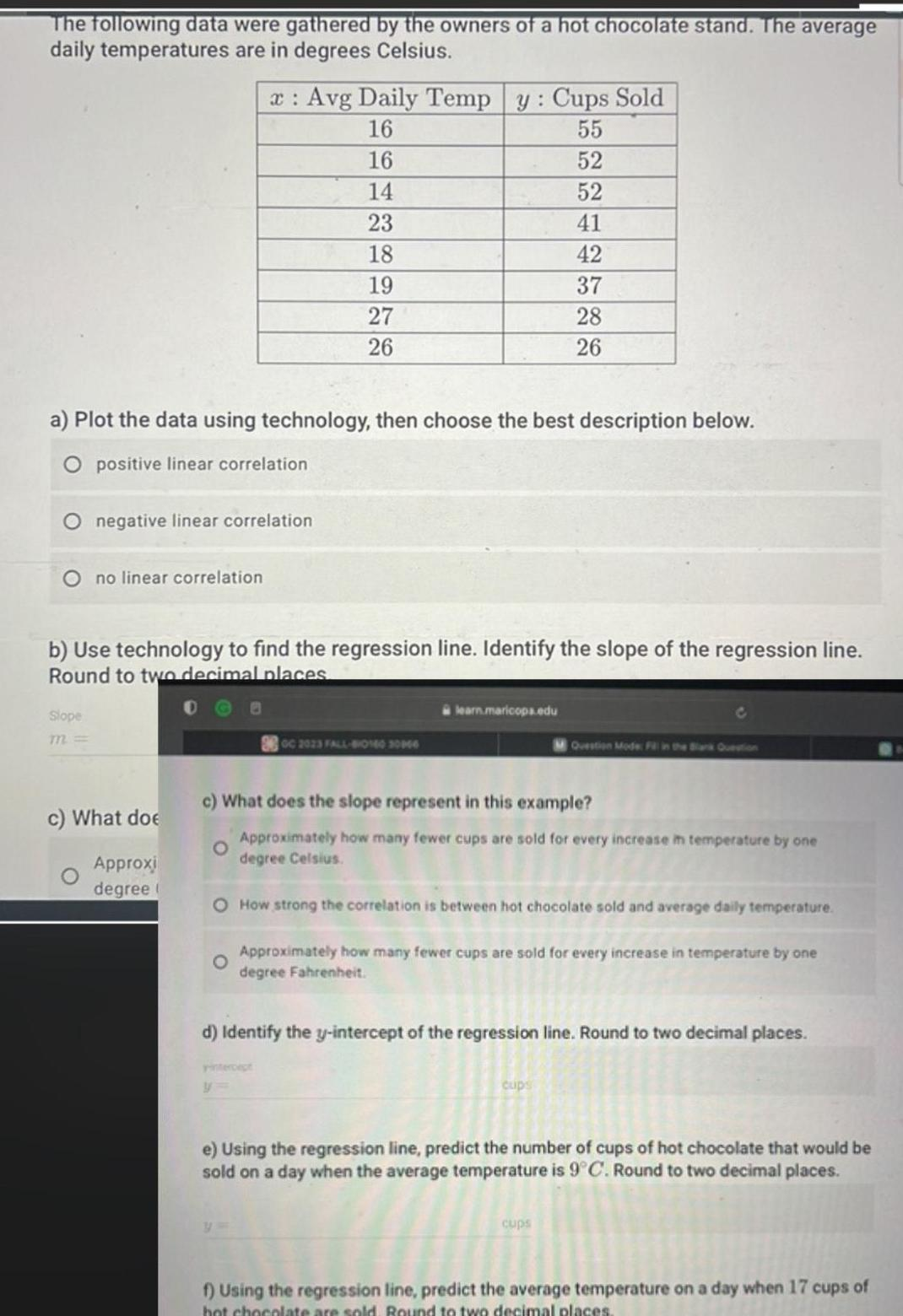Question:

# The following data were gathered by the owners of a hot

Last updated: 9/18/2023The following data were gathered by the owners of a hot chocolate stand The average daily temperatures are in degrees Celsius a Plot the data using technology then choose the best description below O positive linear correlation Slope m negative linear correlation no linear correlation x Avg Daily Temp 16 16 14 23 18 19 27 26 b Use technology to find the regression line Identify the slope of the regression line Round to two decimal places c What doe Approxi degree y Cups Sold 55 52 52 41 42 37 28 26 GC 2023 FALL 014030966 y learn maricopa edu c What does the slope represent in this example O Approximately how many fewer cups are sold for every increase in temperature by one degree Celsius O How strong the correlation is between hot chocolate sold and average daily temperature O Approximately how many fewer cups are sold for every increase in temperature by one degree Fahrenheit d Identify the y intercept of the regression line Round to two decimal places M Question Mode F in the Bank Question cups e Using the regression line predict the number of cups of hot chocolate that would be sold on a day when the average temperature is 9 C Round to two decimal places cups f Using the regression line predict the average temperature on a day when 17 cups of hot chocolate are sold Round to two decimal places